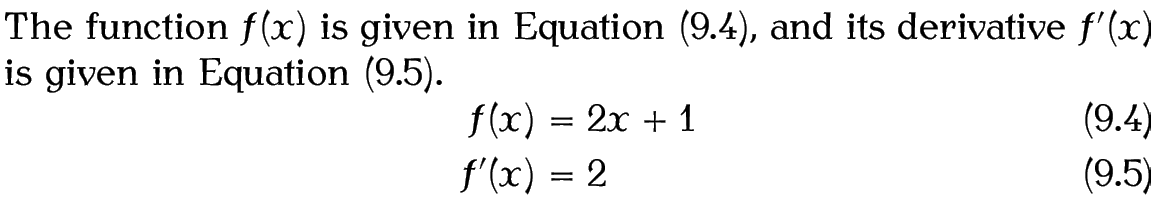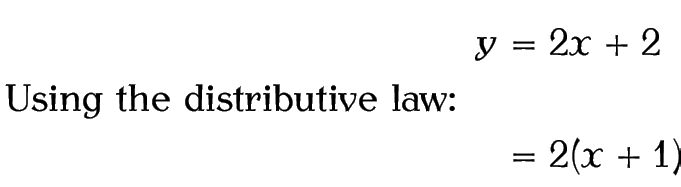# 9.3 Multiple Lines of Displayed Maths

The amsmath package provides the align and align* environments for aligned equations. The starred version doesn't number the equations. These environments provide pairs of left- and right-aligned columns. As with the tabular environment, use & to separate columns and \\ to separate rows. Unlike the tabular environment, there is no argument as the column specifiers are predefined. Another difference is that no page breaks can occur in the tabular environment, but it's possible to allow a page break in align or align* using

immediately before the \\ where it is to take effect. The optional argument is a number from 0 to 4 indicating the desirability to break the page (from 0 the least to 4 the most).

If you want to mix numbered and unnumbered rows, you can use

to suppress the numbering for a particular row in the align environment. This command must go before \\ at the end of the row. The default equation numbering can be overridden for a particular row using:

\tag{<tag>}

where <tag> is the replacement for the equation number.

Don't use the eqnarray or eqnarray* environments. They're obsolete .

Example (Unnumbered):

\begin{align*}
y &= 2x + 2\\
&= 2(x+1)
\end{align*}Note that the equals sign is placed at the start of the second column, after the ampersand &. This ensures the correct amount of spacing on either side. If the first line of the above equation was changed to:

y =& 2x + 2\\
there wouldn't be enough space on the right of the equal sign:Example (One Row Numbered):

\begin{align}
y &= 2x + 2\notag\\
&= 2(x+1)
\end{align}Example (Four Columns):

\begin{align*}
y &= 2x + 2 & z &= 6x + 3\\
&= 2(x+1) &   &= 3(2x+1)
\end{align*}As with equation, you can cross-reference individual rows of an align environment, but you must remember to put \label before the end of row \\ separator. You can reference a row in the align* environment if you have assigned it a tag with \tag, but don't try labelling a row in the align environment where the numbering has been suppressed with \notag.

Example (Cross-Referenced):

This example has two numbered equations in an align environment, both of which are labelled and referenced:

The function $f(x)$ is given in Equation~\eqref{eq:fx}, and its derivative $f'(x)$ is given in Equation~\eqref{eq:dfx}.
\begin{align}
f(x) &= 2x + 1 \label{eq:fx}\\
f'(x) &= 2 \label{eq:dfx}
\end{align}Recall the command \text{<text>} from the previous section. This can be used within cells of the align and align* environments, but the amsmath package also provides

\intertext{<text>}

which can be used for a line of interjection between the rows. This command may only go right after \\.

Example

\begin{align*}
y &= 2x + 2\\
\intertext{Using the distributive law:}
&= 2(x+1)
\end{align*}There are other environments for multiple-line displayed maths, but they are beyond the scope of this book. See the amsmath documentation for further details.

This book is also available as A4 PDF or 12.8cm x 9.6cm PDF or paperback (ISBN 978-1-909440-00-5).

© 2012 Dickimaw Books. "Dickimaw", "Dickimaw Books" and the Dickimaw parrot logo are trademarks. The Dickimaw parrot was painted by Magdalene Pritchett.